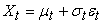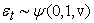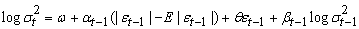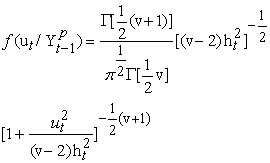﻿ Modeling Volatility under Normal and Student-t Distributional Assumptions (A Case Study of the Kenyan Exchange Rates)

### Modeling Volatility under Normal and Student-t Distributional Assumptions (A Case Study of the Kenya...

Rotich Titus KipkoechOPEN ACCESSPEER-REVIEWED

## Modeling Volatility under Normal and Student-t Distributional Assumptions (A Case Study of the Kenyan Exchange Rates)

Rotich Titus KipkoechDepartment of Mathematics & Computer Science, University of Eldoret, Eldoret, Kenya

### Abstract

The predictive performance of two EGARCH{i} models for modeling daily changes in logarithmic exchange rates (log Rt{ii}) are analyzed here. One is based on modeling the data on assumption of normal distribution and the other is based on the standardized student-t distribution. In particular, the (log Rt) of USDKES{iii}, EUROKES{iv} and GBPKES{v} are considered. For each assumption EGARCH is fitted, with varying numbers of parameters, and attempt to replicate the empirical (log Rt) sequence via simulation. Assessing the fit of each model, it is concluded that the families of EGARCH models with t-innovations adequately reflect the empirical nature of the (log Rt) sequence and therefore provides a better prediction model.

### At a glance: Figures

12
Prev Next

• Kipkoech, Rotich Titus. "Modeling Volatility under Normal and Student-t Distributional Assumptions (A Case Study of the Kenyan Exchange Rates)." American Journal of Applied Mathematics and Statistics 2.4 (2014): 179-184.
• Kipkoech, R. T. (2014). Modeling Volatility under Normal and Student-t Distributional Assumptions (A Case Study of the Kenyan Exchange Rates). American Journal of Applied Mathematics and Statistics, 2(4), 179-184.
• Kipkoech, Rotich Titus. "Modeling Volatility under Normal and Student-t Distributional Assumptions (A Case Study of the Kenyan Exchange Rates)." American Journal of Applied Mathematics and Statistics 2, no. 4 (2014): 179-184.

 Import into BibTeX Import into EndNote Import into RefMan Import into RefWorks

### 1. Introduction

 introduced the concept of modelling volatility based on heteroscedasticity, and since then, several formulations of conditionally heteroskedastic models (for example GARCH{vi} and EGARCH) have been introduced in the literature, a good example being the survey by . One of the earliest asymmetric GARCH models is the EGARCH (Exponential generalized ARCH{vii}) model of . From these studies it has been found that a practical statistical model in finance must be able to capture some stylized facts of financial returns which includes non stationarity of price series, absence of autocorrelation for the price variations, autocorrelations of the squared price returns, volatility clustering, fat-tailed distributions, leverage effects and seasonality.

Although the EGARCH Model is quite successful when predicting price returns, it is known to have certain biases associated with it. There has been strong evidence that financial time series data is heavy tailed as opposed to the normal distribution and that this assumption of a normal distribution creates biases when using the EGARCH model.

Different models have been proven to perform better with heavy tailed assumptions than normal distribution when modelling financial data. This paper adds to this research by comparing the conventional EGARCH model with an EGARCH model re-estimated with a Student t distribution. It aims to show that when data is modeled with EGARCH that allows for t-innovations, it produces better results in terms of predictions as compared to the usual normal distribution as it captures the fat tailed nature of distribution of returns.

GARCH models are helpful if we model the time-varying volatility of the financial assets, ( and ). These models are advantageous because they are easy to estimate and allow us to perform diagnostic tests, see .

 in their research on modeling the volatility of exchange rates concluded GARCH (1, 1){viii} was applicable in estimation of volatility in the Kenyan Foreign exchange market. However GARCH (1, 1) model has a drawback in that it only captures some of the skewness and fat tails in the financial data, . Also, the normal GARCH (1, 1) process fail to forecast financial data with non-normal conditional densities. Therefore, more research has continued in order to find other distributional functions for the error term in order to provide a better explanation of data.

This has led to the introduction of other non-normal conditional densities in the GARCH framework. In particular, the Student t-GARCH models . For data with non-normal conditional variance, the normal GARCH model could not explain the entire fat tail nature of data and it was better to apply the non-normal distributions, such as Student t, normal-lognormal distribution, [3, 11] pointed out the evidence of asymmetric responses, suggesting the leverage effect. In response to the weakness of symmetric assumption, He introduced the exponential GARCH (EGARCH) models with a conditional variance formulation that successfully captured asymmetric response in the conditional variance . EGARCH models have shown to be superior as compared to other models that allow for asymmetric conditional variance, see .

Commercial banks, investment bank amongst others hold portfolios of assets that may include stocks, bonds, currencies, and derivatives. Each institution needs to quantify the amount of risk its portfolio may incur in the course of a day, week, month, or year. For example, a bank needs to assess its potential losses in order to set aside enough capital to cover them. Similarly, a company needs to track the value of its assets and any cash flows resulting from losses in its portfolio. An investment fund may want to understand potential losses on its portfolio, not only to allocate its assets better but also to fulfil its obligation to make set payments to investors. In addition, credit-rating and regulatory agencies must be able to assess likely losses on portfolios as well, since they need to set capital requirements and issue credit ratings.

In Kenya there has been increasing need to assess exposure to foreign exchange risks, for example, in the financial year 2013, foreign exchange gain accounted for 41.75% of East African Portland’s profit before tax which shows the significant amount of exposure most companies have to the foreign exchange volatility. Hence this creates the need to calculate Value at risk on investment portfolios. This implies that an establishment of a most fit model would yield a better assessment on the risk established to the portfolio induced by securities volatility.

Studying volatility is important in risk assessment and management. This is because as volatility changes the price of financial instruments also change. Exchange rates change continuously and this affects the profitability of international trading firms. Thus modelling exchange rate volatility is important in currency portfolio management and valuing assets reserves. Firms will face transaction losses if bad models are used. Therefore a practical model for both forecasting and structural interpretation is needed to avoid these losses. These models can help these firms in hedging decisions and also when to take positions in the exchange rates.

Approximately 50% of currency of exposure in most banks in Kenya is attributed to the USDKES, EURKES and GBPKES. This shows that there is need for banks to monitor the movement of these three rates.

1.1. Organization of the Paper

The paper is split up into the following sections: In section two, a review on some empirical models is done including presentations of their mathematical forms. Results are then presented in section three, which includes the various tests done. The results are presented in figures and tables. Based on the results obtained in section three, conclusions are made in the final section, including recommendations.

### 2. Some Empirical Review

2.1. EGARCH model

 studied and introduced the exponential generalized autoregressive conditional heteroskedastic model (EGARCH) on his study on asymmetric volatility modeling. In financial modeling the variance of the time series is always changing over time. The phenomenon is known as heteroscedasticity which makes it difficult to model the first order of the time series. Financial time series also exhibits a phenomenon known as leverage effect and it presents itself in the form of asymmetry in the data.

The EGARCH model which is from the family of GARCH model is able to model the evolution of the variance. Volatility is a measure of risk which is an important factor in any trading decision, hence the importance of modeling the second moment.

The EGARCH model, unlike the GARCH, is able to model leverage effects. This involves additional variables in the model that would imply possibility of negative conditional volatility if GARCH is used. This is not possible unless EGARCH is applied.

2.1.1. General description of EGARCH (1, 1)

Let (Ɛt) be an iid{ix} sequence such that;

E[Ɛt] = 0 and

Var (Ɛt) = 1.

Then (Xt) is said to be an exponential GARCH (EGARCH (1, 1)) process if it satisfies an equation of the form(1)(2)The α parameter represents a magnitude effect or the symmetric effect of the model, the “GARCH” effect. β measures the persistence in conditional volatility.

When β is relatively large, then volatility takes a long time to die out following a crisis in the market, see . This is irrespective of anything happening in the market.

The θ parameter measures the leverage effect. This parameter is important as it allows the EGARCH model to test for asymmetries. If θ = 0, then the model is symmetric. When θ < 0, then positive shocks (good news) generate less volatility than negative shocks (bad news). When θ > 0, it implies that good news is more destabilizing than bad news. The EGARCH (1, 1) model used got a distinctive feature, i.e., conditional variance was modeled to capture the leverage effect of volatility.

2.2. Specification of the error distribution

The form of the error term distribution plays a large role in the estimation of EGARCH model. As the aim of this paper is to test the performance of the model under two assumptions of the error term i.e. normal distribution and student t-distribution, we formulate the EGARCH with the two different functional forms below.

2.2.1 Normal Distribution

Under a standard normality assumption, equation (2) becomes:

Ɛt ~N (0, 1)

The Gaussian EGARCH model consider volatility clustering, but it is not sufficient to account for all the leptokurtosis that appears in the Kenya shilling exchange rates. The number of very high and very low returns observed suggests that a fatter-tailed distribution might better characterize the error process for Kenya shilling exchange rate returns.

2.2.2. Student t-distribution

The student t-EGARCH model assumes that the conditional distribution of market shocks is t distributed. As observed, the data tends to be heavy tailed and thus the student t-distribution better characterizes the error process. Thus the equation (2) for this case becomes,

Ɛt ~t (0, 1, v)

With mean 0, variance 1 and v degrees of freedom.

In view of the fact that the Gaussian GARCH model could not explain the leptokurtosis exhibited by asset returns,  suggested replacing the assumption of conditional Normality of the error with that of Conditional Student’s t distribution. He argued that this formulation would permit us to distinguish between conditional leptokurtosis and conditional heteroskedasticity as plausible causes of the unconditional kurtosis observed in the data. The distribution of the error term according to  takes the form:### 3. Empirical Results

The data used in this analysis is based on USDKES, GBPKES and EURKES exchange rates which were obtained from the CBK website. R.gui software was used for data analysis.

Figure 1 shows plots of price returns of the three series in that interval. The plots demonstrate the trend of the returns over the past decade and give some intuition as to why these particular intervals were chosen.

All the series show volatility clustering where periods of high volatility are followed by periods of low volatility and vice versa. In all the series, these changes occur at the same periods. Of importance are the periods of high volatility, between 2007 and 2008{1} and between 2011 and the beginning of 2012, this was the period before Kenya’s last election and its incursion to Somalia. These kind of fluctuations maybe difficult to capture by a normal distribution and thus the predictions would be inaccurate, because the normal distribution doesn’t account for large variations in data.

Table 1 presents some sample distributional statistics for the exchange rates studied in this paper. Statistics consist of the daily sample mean returns, standard deviation, minimum returns and maximum returns.

#### Table 1. Distributional characteristics of USDKES, EUROKES and GBPKES

The table below is the correlation matrix of the series.

The pound clearly outperformed the other pairs in the last decade with a positive return. The Euro and the dollar both had negative returns, implying that in the past decade Kenyan shilling had performed better than the dollar and the euro but the pound still remained stronger. Therefore an investor would have profited by holding long KSH against the dollar and the euro and going long on the pound.

The Euro has been highly volatile in the past decade, followed closely by the pound and the dollar being the lowest. This is because in the past decade the euro economy has been facing major crisis recently being the Greece debt crisis.

3.1. Normality test

Normality of the data was tested with two methods. First, the quantiles of normal distribution were obtained. Figure 2 represents the distribution plots for the three series. If the sample is perfectly normally distributed the points should all fall on the 45 degree line. The more the points diverge from this line, the less data will be approximate to a normal distribution. Apparently, all three exchange rate returns do not follow normal distribution in the long run. More points fall on the 45 degree lines in GBPKES and EUROKES, implying that they are perhaps more close to normal distributions whereas USDKES shows low probability to be normal distribution.

Next the skewness and kurtosis of the three series were estimated and D Agastino and Anscombe tests for skewness and kurtosis respectively were utilized to test the significance of the coefficients, whose null is that the sample data are normally distributed.

The coefficients are presented in the table below.

#### Table 3. Skewness and Kurtosis of the returns

It can be observed that coefficients of skewness are not different from one, indicating slight skewness. EUROKES displays a negative skew implying that negative returns are more expected than positive ones, the other two are positively skewed implying the opposite of EURO. On the other hand the kurtosis of all the series are much greater than three, indicating a high level of peakedness. USDKES has the greatest kurtosis implying that it is more heavy tailed than the rest.

The results of the test are presented below.

#### Table 4. D Agastino skewness test and Anscombe kurtosis tests

The null hypothesis that the sample is normally distributed at 5% significant level is rejected. The skewness parameters are highly significant, indicating that the stock market returns were not symmetrically distributed.

Coefficients of kurtosis are all significant in all exchange rates, referring that exchange rate return volatility exist in all exchanges.

3.2. Testing for Autocorrelation

It can be observed that the autocorrelations for the returns are often outside the standard significance levels, which leads to rejection of the strong white noise assumption for all these series. On the other hand, most of the autocorrelations are inside the significance bands shown as solid lines, which is in accordance with the hypothesis that the series are realizations of semi-strong white noises. This is evidence of semi strong form of market hypothesis. In an efficient market the combined effect of information coming in a random, independent, unpredictable fashion and numerous competing investors adjusting market prices rapidly to reflect this new information means that one would expect price changes to be independent and random. However this is not the case with the Kenyan financial market as the players are not many, mostly consisting of large institutions hence the market is prone to manipulation.

From the above figures it can be observed that most autocorrelations of USDKES are outside the significance bands more than the other two series. This shows that the pair does not exhibit much efficiency implying that it’s more manipulated than the rest. It is the claim of this paper that this is so because it’s the major exchange rate and hence the government and large market players have a major interest in it.

The table below shows the p-values of the Ljung Box test for autocorrelations.

#### Table 5. Ljung-Box test for autocorrelation at lag 1

The p-values of the squared returns are very small, indicating that at least one of the first five autocorrelations is nonzero. On the other hand the returns have large p-values which indicates that the null hypothesis that the returns are uncorrelated, at least at small lags should be accepted. However the p-value of USDKES is relatively small than the pound and Euro, showing that its autocorrelations are more prominent. This shows weak form of white noise and hence weak market efficiency in that pair.

This test confirms the analysis from the ACF plots of both the returns and their squares. Hence the data can be modeled using the GARCH family of models.

### 4. Conclusion

This paper has considered the modeling of the exchange rate returns during the last decade under two distributional assumptions.

Exchange rate return data for the three major exchange rates in Kenya have been examined to compare the EGARCH model under the two distributional assumptions: normal and student-t distributions. There are enough evidences to reject the assumptions of normality in the three exchange rates: this is reflected in the form of skewness and kurtosis and the distributional plots. Although traditional EGARCH modeling has been found useful to account for commonly observed stylized characteristics in our sample data, such as skewness, conditional heteroscedasticity, it cannot easily accommodate leptokurtic nature of financial data.

In addition, the evidences of leverage effect and volatility clustering have been observed in the three exchange rates. The results indicate that it is important to specify an EGARCH model which is sufficiently flexible to accommodate these data characteristics because estimation procedures that fail to account for data characteristics are likely to lead to inaccurate results.

The paper finds that the most empirical evidence favors student t EGARCH model which allows for the increased flexibility. Empirical evidences suggest that this EGARCH model provides a better description and a better fit than the traditional EGARCH model with normal distribution. Both GBPKES and EURKES were fitted by an EGARCH (1, 1) while USDKES was fit by an AR (1)/EGARCH (1, 1) with εt ~t (0, 1, v).

Since the EGARCH model with student-t distribution outperforms the normal EGARCH model in terms of structural properties, evaluation of the market was done for the past decade with this model. The findings are; it takes a long time for investors to recover their confidence to market after a financial crisis. Also because Kenya financial markets are still developing, the Kenyan exchange rates exhibit a phenomenon whereby, good news produces much volatility than bad news. This is unlike exchange rates of developed economies. Lastly the dollar is the most sensitive currency to volatility among the three. This is due to the dollar being the most traded exchange rate hence a lot of interest and susceptibility to reacting to market phenomenon.

### References

  Alexakis, P., & Xanthakis, M. (1995). Day-of-the-week effect on the greek stock market. Applied Financial Economics, 5, 43-50.In article CrossRef  Alexander, C. (2009). Practical Financial Econometrics. John Wiley & Sons Ltd.In article  Alexander, C., & Lazar, E. (2006). Normal Mixture GARCH (1,1): Application to exchange rate modeling. Journal of Applied Econometrics Economic Review, 39, 885-905.In article  Bollerslev, T. (1986). A generalized autoregressive conditional heteroscedasticity. Journal of Econometrics, 31, 307-327.In article CrossRef  Bollerslev, T. (1987). A conditional heteroskedastic time series model for speculative rices and rates of return. review of economics and statistics, 69, 542-547.In article CrossRef  Chang, S. (2012). Application of EGARCH model to estimate financial volatility of daily returns: The empirical case of china.In article  Drakos, A. A., Kouretas, G. P., & Zarangas, L. P. (2010). Forecasting financial volatility of the athens stock exchange daily returns: an application of the assymetric normal mixture GARCH model. International Journal of Finance and Economics, 1-4.In article  Engle, R. F. (1982). Autoregressive conditional heteroskedasticity with estimates of the variance of United Kingdom inflation. Econometrica, 50(4), 987–1007.In article CrossRef  Engle, R. F., Bollerslev, T., & Nelson, D. B. (1994). Arch Models. Amsterdam: Handbook of Econometrics.In article  Maana, I., Mwita, P. N., & Odhiambo, R. (2010). Modelling the volatility of exchange rates in the kenyan market. African Journal of Business Management, 4(7), 1401-1408.In article  Nelson, D. B. (1991). Conditional heteroscedasticity in asset returns: a new approach. Econometrica, 59, 347-70.In article CrossRef

### Notes

1Exponential Generalized Autoregressive Conditional Heteroskedastic

2Logarithmic Exchange Rates

3United States Dollar verses Kenyan Shilling

4European Euro verses Kenyan Shilling

5Great Britain Pound verses Kenyan Shilling

6Generalized Autoregressive Conditional Heteroskedastic

7Autoregressive Conditional Heteroskedastic

8GARCH of order (1,1)

9Independently and Identically Distributed

10period is during the world economic crisis and the turbulent election period